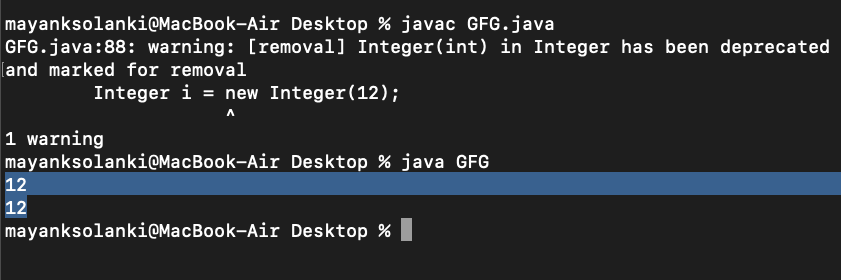Open In App

# Primitive Wrapper Classes are Immutable in Java

In Java, an immutable class is a class (Integer, Byte, Long, Float, Double, Character, Boolean, and Short) which once created then its body can not be changed and the same applies to immutable objects which once created cannot be changed. Now the question arises that we do need the help of wrapper classes while dealing with primitive data types in Java where these classes are made immutable.

Geeks, now you must be wondering why do we make them as immutable for which we will be listing some advantages as listed below as follows:

• They are automatically synchronized as their state can not be altered by virtue of the definition of immutability.
• There are zero scopes of inconsistency as an object of the wrapper class can not be altered.
• It helps in caching as one instance of a specific type itself can facilitate dozen of applications.

Tip: Best usage of wrapper classes as immutable objects is as keys of the Map.

Example:

## Java

 `// Java Program to Demonstrate that Primitive``// Wrapper Classes are Immutable` `// Main class``class` `GFG {` `    ``// Main driver method``    ``public` `static` `void` `main(String[] args)``    ``{` `        ``// Getting an integer value``        ``Integer i = ``new` `Integer(``12``);` `        ``// Printing the same integer value``        ``System.out.println(i);` `        ``// Calling method 2``        ``modify(i);` `        ``// Now printing the value stored in above integer``        ``System.out.println(i);``    ``}` `    ``// Method 2``    ``// To modify integer value``    ``private` `static` `void` `modify(Integer i) { i = i + ``1``; }``}`

Output:Output explanation:

Here the parameter ‘i’ is the reference in modifying and refers to the same object as ‘i’ in main(), but changes made to ‘i’ are not reflected in main() method. It is because all primitive wrapper classes (Integer, Byte, Long, Float, Double, Character, Boolean, and Short) are immutable in Java, so operations like addition and subtraction create a new object and not modify the old.
The below line of code in the modify method is operating on wrapper class Integer, not an int, and does the following as described below as follows:

`i = i + 1;`
• Unbox ‘i’ to an int value
• Add 1 to that value
• Box the result into another Integer object
• Assign the resulting Integer to ‘i’ (thus changing what object ‘i’ references)

Since object references are passed by value, the action taken in the modified method does not change i that was used as an argument in the call to modify. Thus the main routine still prints 12 after the method returns.